## eScience Lectures Notes : Circle drawing algorithm

Slide 1 : 1 / 8 : Line-Drawing Algorithms

# Circle-Drawing Algorithms

## Introduction

### MidPoint algorithm (2)

CircleDraw: a case study...

Slide 2 : 2 / 8 : Circle-Drawing Algorithms

# Circle-Drawing Algorithms

### Beginning with the equation of a circle: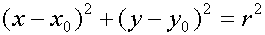### We could solve for y in terms of x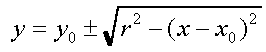,

### and use this equation to compute the pixels of the circle. When finished we'd end up with code that looked something like the following:

```    public void circleSimple(int xCenter, int yCenter, int radius, Color c)
{
int pix = c.getRGB();
int x, y, r2;

y = (int) (Math.sqrt(r2 - x*x) + 0.5);
raster.setPixel(pix, xCenter + x, yCenter + y);
raster.setPixel(pix, xCenter + x, yCenter - y);
}
}```

Slide 3 : 3 / 8 : Let's use 2 symetries : Symetry 4

# Let's use 2 symmetries : Symmetry 4

###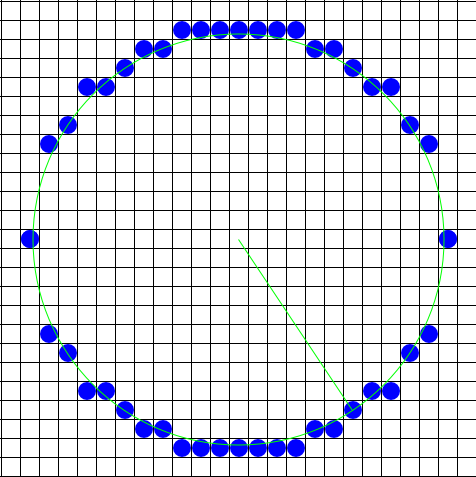Symmetry 2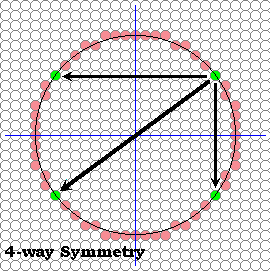### Already : two pixels for each function evaluation

As you can see the circles look fine in areas where only one pixel is required for each column, but in areas of the circle where the local slope is greater the one the circle appears discontinuous (where have we seen this before?).

We could take the approach of computing the derivative (i.e. the local slope) of the function at each point and then make a decision whether to step in the x direction or the y direction. But, we will explore a different approach here.

A circle exhibits a great deal of symmetry. We've already exploited this somewhat by plotting two pixels for each function evaluation; one for each possible sign of the square-root function. This symmetry was about the x-axis. The reason that a square-root function brings out this symmetry results from our predilection that the x-axis should be used as an independent variable in function evaluations while the y-axis is dependent. Thus, since a function can yield only one value per member of the domain, we are forced to make a choice between positive and negative square roots. The net result is that our simple circle-drawing algorithm exploits 2-way symmetry about the x-axis.

Obviously, a circle has a great deal more symmetry. Just as every point above an x-axis drawn through a circle's centre has a symmetric point an equal distance from, but on the other side of the x-axis, each point also has a symmetric point on the opposite side of a y-axis drawn through the circle's centre.

Slide 4 : 4 / 8 : 4-way symmetry algorithm

# 4-way symmetry algorithm

### We can quickly modify our previous algorithm to take advantage of this fact as shown below.

```    public void circleSym4(int xCenter, int yCenter, int radius, Color c)
{
int pix = c.getRGB();
int x, y, r2;

for (x = 1; x <= radius; x++) {
y = (int) (Math.sqrt(r2 - x*x) + 0.5);
raster.setPixel(pix, xCenter + x, yCenter + y);
raster.setPixel(pix, xCenter + x, yCenter - y);
raster.setPixel(pix, xCenter - x, yCenter + y);
raster.setPixel(pix, xCenter - x, yCenter - y);
}
}```

###4-way symmetry algorithm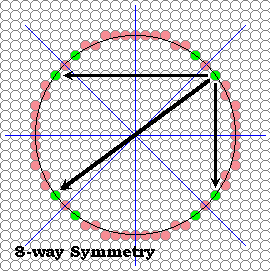This circle-drawing algorithm uses 4-way symmetry.

This algorithm has all the problems of our previous algorithm, but it gives the same result with half as many function evaluations. So much for "making it work first" before optimizing. But, we're on a roll so let's push this symmetry thing as far as it will take us.

Slide 5 : 5 / 8 : 8-way symmetry algorithm

# 8-way symmetry algorithm

### Symmetry about the pair of lines with slopes of one and minus oneWe can find any point's symmetric complement about these lines by permuting the indices. For example the point (x,y) has a complementary point (y,x) about the line x=y. And the total set of complements for the point (x,y) are

### algorithm loops over one-eighth of the circle from the top to the right by 45 degrees

The following routine takes advantage of this 8-way symmetry. The algorithm loops over one-eighth of the circle. Specifically, it starts at the top of the circle and goes to the right by 45 degrees, where the slope of a radial line is 1. Thus, the stopping state is crossing the x=y line.

```    public void circleSym8(int xCenter, int yCenter, int radius, Color c)
{
int pix = c.getRGB();
int x, y, r2;

x = 1;
y = (int) (Math.sqrt(r2 - 1) + 0.5);
while (x < y) {
raster.setPixel(pix, xCenter + x, yCenter + y);
raster.setPixel(pix, xCenter + x, yCenter - y);
raster.setPixel(pix, xCenter - x, yCenter + y);
raster.setPixel(pix, xCenter - x, yCenter - y);
raster.setPixel(pix, xCenter + y, yCenter + x);
raster.setPixel(pix, xCenter + y, yCenter - x);
raster.setPixel(pix, xCenter - y, yCenter + x);
raster.setPixel(pix, xCenter - y, yCenter - x);
x += 1;
y = (int) (Math.sqrt(r2 - x*x) + 0.5);
}
if (x == y) {
raster.setPixel(pix, xCenter + x, yCenter + y);
raster.setPixel(pix, xCenter + x, yCenter - y);
raster.setPixel(pix, xCenter - x, yCenter + y);
raster.setPixel(pix, xCenter - x, yCenter - y);
}
}```

### Routine should be approximately 4-times faster than our initial circle-drawing algorithm

So now we get 8 points for every function evaluation, and this routine should be approximately 4-times faster than our initial circle-drawing algorithm. What's going on with the four pixels that are set outside the loop (both at the top and bottom)? Didn't I say that every point determines 7 others?

## And It Works ! Without any special code to test for the slope ??? Not Really

It seems suddenly that our circles appear continuous, and we added no special code to test for the slope. Symmetry has come to our rescue. (Actually, symmetry is also what saved us on lines, if you think about it.)

Slide 6 : 6 / 8 : 8-way symmetry algorithm

# MidPoint algorithm

### Let's simplify the function evaluation that takes place on each iteration of our circle-drawing algorithm

So our next objective is to simplify the function evaluation that takes place on each iteration of our circle-drawing algorithm. All those multiplies and square-root evaluations are expensive. We can do better.

### We translate our coordinate system so that the circle's center is at the origin

One approach is to manipulate the circle equation slightly. First, we translate our coordinate system so that the circle's center is at the origin (the book leaves out this step), giving:

### We simplify and make the equation homogeneous

Next, we simplify and make the equation homogeneous (i.e. independent of a scaling of the independent variables; making the whole equation equal to zero will accomplish this) by subtracting r2 from both sides.

### x2 + y2 - r2 = 0

We can regard this expression as a function in x and y.

## f( x, y ) = x2 + y2 - r2

Functions of this sort are called discriminating functions in computer graphics. They have the property of partitioning the domain, pixel coordinates in our case, into one of three categories. When f(x,y) is equal to zero the point lies on the desired locus (a circle in this case), when f(x, y) evaluates to a positive result the point lies one side of the locus, and when f(x,y) evaluates to negative it lies on the other side.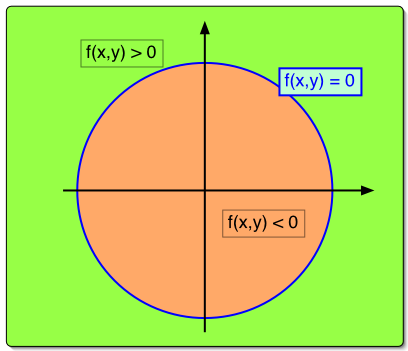Slide 7 : 7 / 8 : MidPoint algorithm (2)

# MidPoint algorithm (2)

What we'd like to do is to use this discriminating function to maintain our trajectory of drawn pixels as close as possible to the desired circle. Luckily, we can start with a point on the circle, (x0, y0+r) (or (0, r) in our adjusted coordinate system). As we move along in steps of x we note that the slope is less than zero and greater than negative one at points in the direction we're heading that are near our known point on a circle. Thus we need only to figure out at each step whether to step down in y or maintain y at each step.

• ### M ouside (f>0) => next point is SE### D0 = f(1, r-1/2) = 5/4 - r ...not an inger Lets change every where D by P = D - 1/4 P0 = 1 - r , and because increment are always integer, P < -1/4 <=> P < 0

```        int x = 0;        int y = radius;
int p = 1 - radius;        circlePoints(xCenter, yCenter, x, y, pix);        while (x < y) {            x++;            if (p < 0) {                p += 2*x+1;            } else {                y--;                p += 2*(x-y+1);            }            circlePoints(xCenter, yCenter, x, y, pix);        } ```